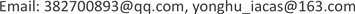1北京中关村永丰产业基地发展有限公司，北京

2北京寰宇声望智能科技有限公司，北京1. 引言

2. 振动信号目标特征提取及识别算法

2.1. Fourier分析

F ( ω ) = ∫ − ∞ + ∞ x ( t ) e − i ω t d t (1)

x ( t ) = 1 2 π ∫ − ∞ + ∞ F ( ω ) e i ω t d ω (2)

2.2. 小波分析

ψ a τ ( t ) = 1 a ψ ( t − τ a ) (3)

W T x ( a , τ ) = 1 a ∫ x ( t ) ψ * ( t − τ a ) d t (4)

2.3. 主成分分析

w ^ = arg min ‖ w ‖ 2 = 1 w T X T X w (5)

2.4. 支持向量机

w ∗ x + b = 0 (6)

y i [ w ∗ x i + b ] ≥ 1 − ξ i ,   i = 1 , 2... , n ,   ξ i ≥ 0 (7)

w ^ = arg min w 1 2 ‖ w ‖ 2 + C ∑ i = 1 n ξ i (8)

f ( x ) = s i g n ( ∑ i = 1 n y i α i ∗ ( x i ∗ x ) + b ∗ ) (9)

3. 振动信号目标识别实验3.1. 样本说明

Sample informatio

Healthy20Hz1042 × 10,000
Healthy40Hz1021 × 10,000
YangCo20HZ1027 × 10,000
Yangpa40HZ1030 × 10,000
YangUn20HZ1037 × 10,000
YangUn40HZ1029 × 10,000

Feature extraction and classifier descriptio

FFT快速傅里叶变换
PCA主成分分析
WT小波变换
LibLinSvmSVM线性分类器

3.2. 实验结果分析

10次平均结果，测试总体分类正确率及每类样本分类正确率如表3所示：

Comparison of classification result

CH10.998110.99610.9921
CH20.995110.99510.9931
CH30.988110.9960.9610.9920.984
CH40.839110.8530.8930.8280.812

4. 总结